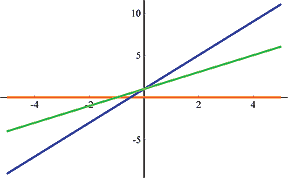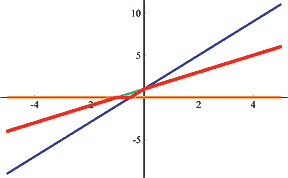Online JudgeProblem SetAuthorsOnline ContestsUser
Web Board
F.A.Qs
Statistical Charts
Problems
Submit Problem
Online Status
Prob.ID:
Register
Authors ranklist
Current Contest
Past Contests
Scheduled Contests
Award Contest
Register
Language:
Median of Lines
 Time Limit: 6000MS Memory Limit: 131072K Total Submissions: 1504 Accepted: 311

Description

In the Cartesian plane, there are n (odd) distinct lines fi(x) = ai + xbi (i = 1, 2, …, n). For each x, F(x) denotes the median of {f1(x), f2(x), ..., fn(x)}. You are required to find the solution space of the equation F(x) = 0.

Input

The input contains multiple test cases. Each test case have n + 1 lines the first one of which contains n (1 < n < 105 and odd). Then n lines follow, each of which contains two integers ai and bi (|ai| ≤ 108, 0 ≤ bi < 108). A zero follows the last test case.

Output

For each test case, output the solution space as an interval on a separate line. Interval boundaries should be rounded to two digits beyond the decimal point. “`+inf`” and “`-inf`” are used to represent positive and negative infinities. The solution space will form at most one interval in this problem. If the solution space is empty, just output “`-1`”.

Sample Input

```3
0 0
1 0
0 1
3
0 0
1 2
1 1
3
1 0
2 0
3 0
3
1 1
1 2
1 3
3
0 0
1 0
-1 0
0```

Sample Output

```(-inf,0.00]
[-1.00,-0.50]
-1
[-0.50,-0.50]
(-inf,+inf)```

Hint

Be cautious about outputting “`-0.00`”.

Illustration of the second test case in the sample input:Source

[Submit]   [Go Back]   [Status]   [Discuss]Home PageGo BackTo top